Theory and Modern Applications

# LMI approach to robust stability and stabilization of nonlinear uncertain discrete-time systems with convex polytopic uncertainties

## Abstract

This article addresses the robust stability for a class of nonlinear uncertain discrete-time systems with convex polytopic of uncertainties. The system to be considered is subject to both interval time-varying delays and convex polytopic-type uncertainties. Based on the augmented parameter-dependent Lyapunov-Krasovskii functional, new delay-dependent conditions for the robust stability are established in terms of linear matrix inequalities. An application to robust stabilization of nonlinear uncertain discrete-time control systems is given. Numerical examples are included to illustrate the effectiveness of our results.

MSC:15A09, 52A10, 74M05, 93D05.

## 1 Introduction

Since the time delay is frequently viewed as a source of instability and encountered in various engineering systems such as chemical processes, long transmission lines in pneumatic systems, networked control systems, etc., the study of delay systems has received much attention and various topics have been discussed over the past years. The problem of stability and stabilization of dynamical systems with time delays has received considerable attention, and lots of interesting results have reported in the literature, see  and the references therein. Some delay-dependent stability criteria for discrete-time systems with time-varying delay are investigated in [2, 6, 911], where the discrete Lyapunov functional method are employed to prove stability conditions in terms of linear matrix inequalities (LMIs). A number research works for dealing with asymptotic stability problem for discrete systems with interval time-varying delays have been presented in . Theoretically, stability analysis of the systems with time-varying delays is more complicated, especially for the case where the system matrices belong to some convex polytope. In this case, the parameter-dependent Lyapunov-Krasovskii functionals are constructed as the convex combination of a set of functions assures the robust stability of the nominal systems and the stability conditions must be solved upon a grid on the parameter space, which results in testing a finite number of LMIs [11, 23, 24]. To the best of the authors’ knowledge, the stability for linear discrete-time systems with both time-varying delays and polytopic uncertainties has not been fully investigated. The articles [25, 26] propose sufficient conditions for robust stability of discrete and continuous polytopic systems without time delays. More recently, combining the ideas in [23, 24], improved conditions for $\mathcal{D}$-stability and $\mathcal{D}$-stabilization of linear polytopic delay-difference equations with constant delays have been proposed in .

In this article, we consider polytopic nonlinear uncertain discrete-time equations with interval time-varying delays. Using the parameter-dependent Lyapunov-Krasovskii functional combined with LMI techniques, we propose new criteria for the robust stability of the nonlinear uncertain system. The delay-dependent stability conditions are formulated in terms of LMIs, being thus solvable by the numeric technology available in the literature to date. The result is applied to robust stabilization of nonlinear uncertain discrete-time control systems. Compared to other results, our result has its own advantages. First, it deals with the nonlinear uncertain delay-difference system, where the state-space data belong to the convex polytope of uncertainties and the rate of change of the state depends not only on the current state of the nonlinear systems but also its state at some times in the past. Second, the time-delay is assumed to be a time-varying function belonging to a given interval, which means that the lower and the upper bounds for the time-varying delay are available. Third, our approach allows us to apply in robust stabilization of the nonlinear uncertain discrete-time system subjected to polytopic uncertainties and external controls. Therefore, our results are more general than the related previous results.

The article is organized as follows. In Section 2, introduces the main notations, definitions, and some lemmas needed for the development of the main results. In Section 3, sufficient conditions are derived for robust stability, stabilization of nonlinear uncertain discrete-time systems with interval time-varying delays and polytopic uncertainties. They are followed by some remarks. Illustrative examples are given in Section 4.

## 2 Preliminaries

The following notations will be used throughout this article. ${R}^{+}$ denotes the set of all real non-negative numbers; ${R}^{n}$ denotes the n-dimensional space with the scalar product $〈\cdot ,\cdot 〉$ and the vector norm $\parallel \cdot \parallel$; ${R}^{n×r}$ denotes the space of all matrices of $\left(n×r\right)$-dimension. ${A}^{T}$ denotes the transpose of A; a matrix A is symmetric if $A={A}^{T}$, a matrix I is the identity matrix of appropriate dimension.

Matrix A is semi-positive definite ($A\ge 0$) if $〈Ax,x〉\ge 0$, for all $x\in {R}^{n}$; A is positive definite ($A>0$) if $〈Ax,x〉>0$ for all $x\ne 0$; $A\ge B$ means $A-B\ge 0$.

Consider a nonlinear uncertain delay-difference systems with polytopic uncertainties of the form where $x\left(k\right)\in {R}^{n}$ is the state, the system matrices are subjected to uncertainties and belong to the polytope Ω given by

$\mathrm{\Omega }=\left\{\left[A,D\right]\left(\xi \right):=\sum _{i=1}^{p}{\xi }_{i}\left[{A}_{i},{D}_{i}\right],\sum _{i=1}^{p}{\xi }_{i}=1,{\xi }_{i}\ge 0\right\},$
(2.1)

where ${A}_{i}$, ${D}_{i}$, $i=1,2,\dots ,p$, are given constant matrices with appropriate dimensions.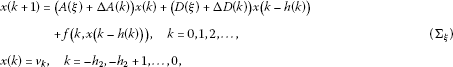The nonlinear perturbations $f\left(k,x\left(k-h\left(k\right)\right)\right)$ satisfies the following condition

${f}^{T}\left(k,x\left(k-h\left(k\right)\right)\right)f\left(k,x\left(k-h\left(k\right)\right)\right)\le {\beta }^{2}{x}^{T}\left(k-h\left(k\right)\right)x\left(k-h\left(k\right)\right),$
(2.2)

where β is positive constants. For simplicity, we denote $f\left(k,x\left(k-h\left(k\right)\right)\right)$ by f, respectively.

The time-varying uncertain matrices $\mathrm{\Delta }A\left(k\right)$ and $\mathrm{\Delta }D\left(k\right)$ are defined by

$\mathrm{\Delta }A\left(k\right)={E}_{a}{F}_{a}\left(k\right){H}_{a},\phantom{\rule{2em}{0ex}}\mathrm{\Delta }D\left(k\right)={E}_{d}{F}_{d}\left(k\right){H}_{d},$
(2.3)

where ${E}_{a}$, ${E}_{d}$, ${H}_{a}$, ${H}_{d}$ are known constant real matrices with appropriate dimensions. ${F}_{a}\left(k\right)$, ${F}_{d}\left(k\right)$ are unknown uncertain matrices satisfying

${F}_{a}^{T}\left(k\right){F}_{a}\left(k\right)\le I,\phantom{\rule{2em}{0ex}}{F}_{d}^{T}\left(k\right){F}_{d}\left(k\right)\le I,\phantom{\rule{1em}{0ex}}k=0,1,2,\dots ,$
(2.4)

where I is the identity matrix of appropriate dimension.

The time-varying function $h\left(k\right)$ satisfies the condition:

$0<{h}_{1}\le h\left(k\right)\le {h}_{2},\phantom{\rule{1em}{0ex}}\mathrm{\forall }k=0,1,2,\dots .$

Remark 2.1 It is worth noting that the time delay is a time-varying function belonging to a given interval, which allows the time-delay to be a fast time-varying function and the lower bound is not restricted to being zero as considered in [2, 6, 911].

Definition 2.1 The nonlinear uncertain system (${\mathrm{\Sigma }}_{\xi }$) is robustly stable if the zero solution of the system is asymptotically stable for all uncertainties which satisfy (2.1), (2.3), and (2.4).

Proposition 2.1 For real numbers${\xi }_{i}\ge 0$, $i=1,2,\dots ,p$, ${\sum }_{i=1}^{p}{\xi }_{i}=1$, the following inequality hold

$\left(p-1\right)\sum _{i=1}^{p}{\xi }_{i}^{2}-2\sum _{i=1}^{p-1}\sum _{j=i+1}^{p}{\xi }_{i}{\xi }_{j}\ge 0.$

Proof The proof is followed from the completing the square:

$\left(p-1\right)\sum _{i=1}^{p}{\xi }_{i}^{2}-2\sum _{i=1}^{p-1}\sum _{j=i+1}^{p}{\xi }_{i}{\xi }_{j}=\sum _{i=1}^{p-1}\sum _{j=i+1}^{p}{\left({\xi }_{i}-{\xi }_{j}\right)}^{2}\ge 0.$

□

Proposition 2.2 (Cauchy inequality)

For any symmetric positive definite matrix $N\in {M}^{n×n}$ and $a,b\in {R}^{n}$ we have

$\underline{+}{a}^{T}b\le {a}^{T}Na+{b}^{T}{N}^{-1}b.$

Proposition 2.3 ()

Let E, H and F be any constant matrices of appropriate dimensions and${F}^{T}F\le I$. For any$ϵ>0$, we have

$EFH+{H}^{T}{F}^{T}{E}^{T}\le ϵE{E}^{T}+{ϵ}^{-1}{H}^{T}H.$

## 3 Main results

### 3.1 Robust stability

In this section, we present sufficient delay-dependent conditions for the robust stability of nonlinear uncertain system (${\mathrm{\Sigma }}_{\xi }$).

Let us set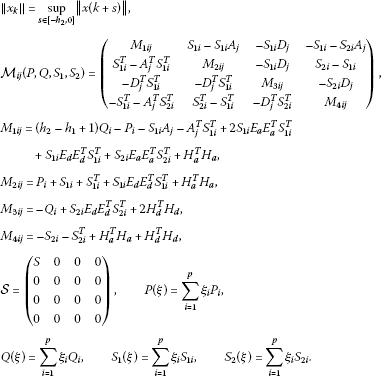Theorem 3.1 The nonlinear uncertain system (${\mathrm{\Sigma }}_{\xi }$) is robustly stable if there exist symmetric matrices${P}_{i}>0$, ${Q}_{i}>0$, $i=1,2\dots ,p$and constant matrices$S\ge 0$, ${S}_{1i}$, ${S}_{2i}$, $i=1,2\dots ,p$satisfying the following LMIs:

1. (i)

${\mathcal{M}}_{ii}\left(P,Q,{S}_{1},{S}_{2}\right)+\mathcal{S}<0$, $i=1,2,\dots ,p$.

2. (ii)

${\mathcal{M}}_{ij}\left(P,Q,{S}_{1},{S}_{2}\right)+{\mathcal{M}}_{ji}\left(P,Q,{S}_{1},{S}_{2}\right)-\frac{2}{p-1}\mathcal{S}<0$, $i=1,2,\dots ,p-1$; $j=i+1,\dots ,p$.

Proof Consider the following parameter-dependent Lyapunov-Krasovskii functional for system (${\mathrm{\Sigma }}_{\xi }$)

$V\left(k\right)={V}_{1}\left(k\right)+{V}_{2}\left(k\right)+{V}_{3}\left(k\right),$

where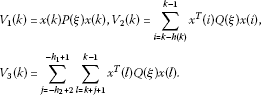We can verify that

${\lambda }_{1}{\parallel x\left(k\right)\parallel }^{2}\le V\left(k\right)\le {\lambda }_{2}{\parallel {x}_{k}\parallel }^{2}.$
(3.1)

Let us set $z\left(k\right)={\left[x\left(k\right)x\left(k+1\right)x\left(k-h\left(k\right)\right)f\left(k,x\left(k-h\left(k\right)\right)\right)\right]}^{T}$, and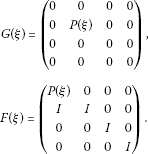Then, the difference of ${V}_{1}\left(k\right)$ along the solution of the system (${\mathrm{\Sigma }}_{\xi }$), we obtained

$\begin{array}{rcl}\mathrm{\Delta }{V}_{1}\left(k\right)& =& {x}^{T}\left(k+1\right)P\left(\xi \right)x\left(k+1\right)-{x}^{T}\left(k\right)P\left(\xi \right)x\left(k\right)\\ =& z{\left(k\right)}^{T}G\left(\xi \right)z\left(k\right)-2{z}^{T}\left(k\right){F}^{T}\left(\xi \right)\left(\begin{array}{c}0.5x\left(k\right)\\ 0\\ 0\\ 0\end{array}\right),\end{array}$
(3.2)

because of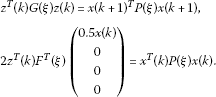Using the expression of system (${\mathrm{\Sigma }}_{\xi }$)

$\begin{array}{rcl}0& =& -{S}_{1}\left(\xi \right)x\left(k+1\right)+{S}_{1}\left(\xi \right)\left(A\left(\xi \right)+\mathrm{\Delta }A\left(k\right)\right)x\left(k\right)\\ +{S}_{1}\left(\xi \right)\left(D\left(\xi \right)+\mathrm{\Delta }D\left(k\right)\right)x\left(k-h\left(k\right)\right)+{S}_{1}\left(\xi \right)f,\\ 0& =& -{S}_{2}\left(\xi \right)x\left(k+1\right)+{S}_{2}\left(\xi \right)\left(A\left(\xi \right)+\mathrm{\Delta }A\left(k\right)\right)x\left(k\right)\\ +{S}_{2}\left(\xi \right)\left(D\left(\xi \right)+\mathrm{\Delta }D\left(k\right)\right)x\left(k-h\left(k\right)\right)+{S}_{2}\left(\xi \right)f,\end{array}$

we have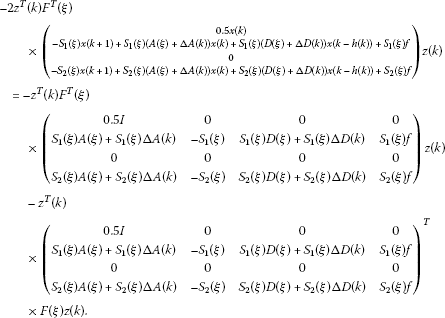Therefore, from (3.2) it follows that

$\begin{array}{rcl}\mathrm{\Delta }{V}_{1}\left(k\right)& =& {x}^{T}\left(k\right)\left[-P\left(\xi \right)-{S}_{1}\left(\xi \right)A\left(\xi \right)-{S}_{1}\left(\xi \right){E}_{a}{F}_{a}\left(k\right){H}_{a}\\ -A{\left(\xi \right)}^{T}{S}_{1}^{T}\left(\xi \right)-{H}_{a}^{T}{F}_{a}^{T}\left(k\right){E}_{a}^{T}{S}_{1}^{T}\left(\xi \right)\right]x\left(k\right)\\ +2{x}^{T}\left(k\right)\left[{S}_{1}\left(\xi \right)-{S}_{1}\left(\xi \right)A\left(\xi \right)-{S}_{1}\left(\xi \right){E}_{a}{F}_{a}\left(k\right){H}_{a}\right]x\left(k+1\right)\\ +2{x}^{T}\left(k\right)\left[-{S}_{1}\left(\xi \right)D\left(\xi \right)-{S}_{1}\left(\xi \right){E}_{d}{F}_{d}\left(k\right){H}_{d}\right]x\left(k-h\left(k\right)\right)\\ +2{x}^{T}\left(k\right)\left[-{S}_{1}\left(\xi \right)-{S}_{2}\left(\xi \right)A\left(\xi \right)-{S}_{2}\left(\xi \right){E}_{a}{F}_{a}\left(k\right){H}_{a}\right]f\left(k,x\left(k-h\left(k\right)\right)\right)\\ +x\left(k+1\right)\left[P\left(\xi \right)+{S}_{1}\left(\xi \right)+{S}_{1}^{T}\left(\xi \right)\right]x\left(k+1\right)\\ +2x\left(k+1\right)\left[-{S}_{1}\left(\xi \right)D\left(\xi \right)-{S}_{1}\left(\xi \right){E}_{d}{F}_{d}\left(k\right){H}_{d}\right]x\left(k-h\left(k\right)\right)\\ +2x\left(k+1\right)\left[{S}_{2}\left(\xi \right)-{S}_{1}\left(\xi \right)\right]f\left(k,x\left(k-h\left(k\right)\right)\right)\\ +2{x}^{T}\left(k-h\left(k\right)\right)\left[-{S}_{2}\left(\xi \right)D\left(\xi \right)-{S}_{2}\left(\xi \right){E}_{d}{F}_{d}\left(k\right){H}_{d}\right]f\left(k,x\left(k-h\left(k\right)\right)\right)\\ +{f}^{T}\left(k,x\left(k-h\left(k\right)\right)\right)\left(k\right)\left[-{S}_{2}-{S}_{2}^{T}\right]f\left(k,x\left(k-h\left(k\right)\right)\right).\end{array}$

Applying Propositions 2.2, 2.3 and condition (2.4), the following estimations hold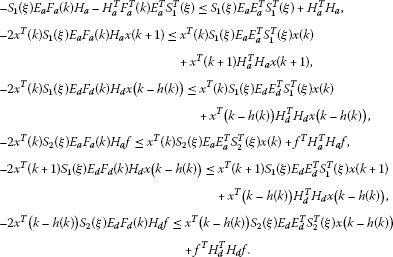Therefore, we have

$\begin{array}{rcl}\mathrm{\Delta }{V}_{1}\left(k\right)& =& {x}^{T}\left(k\right)\left[-P\left(\xi \right)-{S}_{1}\left(\xi \right)A\left(\xi \right)-A{\left(\xi \right)}^{T}{S}_{1}^{T}\left(\xi \right)+2{S}_{1}\left(\xi \right){E}_{a}{E}_{a}^{T}{S}_{1}^{T}\left(\xi \right)\\ +{S}_{1}\left(\xi \right){E}_{d}{E}_{d}^{T}{S}_{1}^{T}\left(\xi \right)+{S}_{2}\left(\xi \right){E}_{a}{E}_{a}^{T}{S}_{2}^{T}\left(\xi \right)+{H}_{a}^{T}{H}_{a}\right]x\left(k\right)\\ +2{x}^{T}\left(k\right)\left[{S}_{1}\left(\xi \right)-{S}_{1}\left(\xi \right)A\left(\xi \right)\right]x\left(k+1\right)\\ +2{x}^{T}\left(k\right)\left[-{S}_{1}\left(\xi \right)D\left(\xi \right)\right]x\left(k-h\left(k\right)\right)\\ +2{x}^{T}\left(k\right)\left[-{S}_{1}\left(\xi \right)-{S}_{2}\left(\xi \right)A\left(\xi \right)\right]f\left(k,x\left(k-h\left(k\right)\right)\right)\\ +x\left(k+1\right)\left[P\left(\xi \right)+{S}_{1}\left(\xi \right)+{S}_{1}^{T}\left(\xi \right)+{S}_{1}\left(\xi \right){E}_{d}{E}_{d}^{T}{S}_{1}^{T}\left(\xi \right)+{H}_{a}^{T}{H}_{a}\right]x\left(k+1\right)\\ +2x\left(k+1\right)\left[-{S}_{1}\left(\xi \right)D\left(\xi \right)\right]x\left(k-h\left(k\right)\right)\\ +2x\left(k+1\right)\left[{S}_{2}\left(\xi \right)-{S}_{1}\left(\xi \right)\right]f\left(k,x\left(k-h\left(k\right)\right)\right)\\ +{x}^{T}\left(k-h\left(k\right)\right)\left[{S}_{2}\left(\xi \right){E}_{d}{E}_{d}^{T}{S}_{2}^{T}\left(\xi \right)+2{H}_{d}^{T}{H}_{d}\right]x\left(k-h\left(k\right)\right)\\ +2{x}^{T}\left(k-h\left(k\right)\right)\left[-{S}_{2}\left(\xi \right)D\left(\xi \right)\right]f\left(k,x\left(k-h\left(k\right)\right)\right)\\ +{f}^{T}\left(k,x\left(k-h\left(k\right)\right)\right)\left(k\right)\left[-{S}_{2}\left(\xi \right)-{S}_{2}^{T}\left(\xi \right)+{H}_{a}^{T}{H}_{a}+{H}_{d}^{T}{H}_{d}\right]\\ ×f\left(k,x\left(k-h\left(k\right)\right)\right).\end{array}$
(3.3)

The difference of ${V}_{2}\left(k\right)$ is given by

$\begin{array}{rcl}\mathrm{\Delta }{V}_{2}\left(k\right)& =& \sum _{i=k+1-h\left(k+1\right)}^{k}{x}^{T}\left(i\right)Q\left(\xi \right)x\left(i\right)-\sum _{i=k-h\left(k\right)}^{k-1}{x}^{T}\left(i\right)Q\left(\xi \right)x\left(i\right)\\ =& \sum _{i=k+1-h\left(k+1\right)}^{k-{h}_{1}}{x}^{T}\left(i\right)Q\left(\xi \right)x\left(i\right)+{x}^{T}\left(k\right)Q\left(\xi \right)x\left(k\right)-{x}^{x}\left(k-h\left(k\right)\right)Q\left(\xi \right)x\left(k-h\left(k\right)\right)\\ +\sum _{i=k+1-{h}_{1}}^{k-1}{x}^{T}\left(i\right)Q\left(\xi \right)x\left(i\right)-\sum _{i=k+1-h\left(k\right)}^{k-1}{x}^{T}\left(i\right)Q\left(\xi \right)x\left(i\right).\end{array}$
(3.4)

Since $h\left(k\right)\ge {h}_{1}$ we have

$\sum _{i=k+1-{h}_{1}}^{k-1}{x}^{T}\left(i\right)Q\left(\xi \right)x\left(i\right)-\sum _{i=k+1-h\left(k\right)}^{k-1}{x}^{T}\left(i\right)Q\left(\xi \right)x\left(i\right)\le 0,$

and hence from (3.4) we have

$\mathrm{\Delta }{V}_{2}\left(k\right)\le \sum _{i=k+1-h\left(k+1\right)}^{k-{h}_{1}}{x}^{T}\left(i\right)Q\left(\xi \right)x\left(i\right)+{x}^{T}\left(k\right)Q\left(\xi \right)x\left(k\right)-{x}^{T}\left(k-h\left(k\right)\right)Q\left(\xi \right)x\left(k-h\left(k\right)\right).$
(3.5)

The difference of ${V}_{3}\left(k\right)$ is given by

$\begin{array}{rcl}\mathrm{\Delta }{V}_{3}\left(k\right)& =& \sum _{j=-{h}_{2}+2}^{-{h}_{1}+1}\left[{x}^{T}\left(k\right)Q\left(\xi \right)x\left(k\right)-{x}^{T}\left(k+j-1\right)Q\left(\xi \right)x\left(k+j-1\right)\right]\\ =& \left({h}_{2}-{h}_{1}\right){x}^{T}\left(k\right)Q\left(\xi \right)x\left(k\right)-\sum _{l=k+1-{h}_{2}}^{k-{h}_{1}}{x}^{T}\left(l\right)Q\left(\xi \right)x\left(l\right).\end{array}$
(3.6)

Since

$\sum _{i=k=1-h\left(k+1\right)}^{k-{h}_{1}}{x}^{T}\left(i\right)Q\left(\xi \right)x\left(i\right)-\sum _{i=k+1-{h}_{2}}^{k-{h}_{1}}{x}^{T}\left(i\right)Q\left(\xi \right)x\left(i\right)\le 0,$

we obtain from (3.5) and (3.6) that

$\mathrm{\Delta }{V}_{2}\left(k\right)+\mathrm{\Delta }{V}_{3}\left(k\right)\le \left({h}_{2}-{h}_{1}+1\right){x}^{T}\left(k\right)Q\left(\xi \right)x\left(k\right)-{x}^{T}\left(k-h\left(k\right)\right)Q\left(\xi \right)x\left(k-h\left(k\right)\right).$
(3.7)

Therefore, combining the inequalities (3.3), (3.7) gives

$\mathrm{\Delta }V\left(k\right)\le {z}^{T}\left(k\right)T\left(\xi \right)z\left(k\right),$
(3.8)

where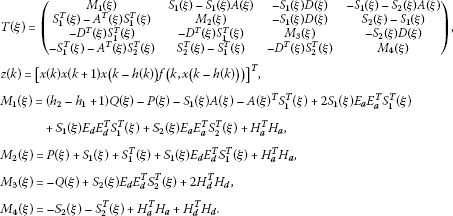Let us denote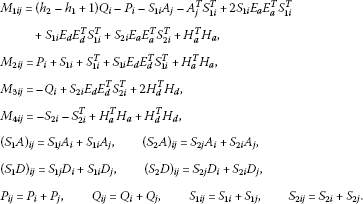From the convex combination of the expression of $P\left(\xi \right)$, $Q\left(\xi \right)$, ${S}_{1}\left(\xi \right)$, ${S}_{2}\left(\xi \right)$, $A\left(\xi \right)$, $D\left(\xi \right)$, we have

$\begin{array}{rcl}T\left(\xi \right)& =& \sum _{i=1}^{p}{\xi }_{i}^{2}\left(\begin{array}{cccc}{M}_{1ii}& {S}_{1i}-{S}_{1i}{A}_{i}& -{S}_{1i}{D}_{i}& -{S}_{1i}-{S}_{2i}{A}_{i}\\ {S}_{1i}^{T}-{A}_{i}^{T}{S}_{1i}^{T}& {M}_{2ii}& -{S}_{1i}{D}_{i}& {S}_{2i}-{S}_{1i}\\ -{D}_{i}^{T}{S}_{1i}^{T}& -{D}_{i}^{T}{S}_{1i}^{T}& {M}_{3ii}& -{S}_{2i}{D}_{i}\\ -{S}_{1i}^{T}-{A}_{i}^{T}{S}_{2i}^{T}& {S}_{2i}^{T}-{S}_{1i}^{T}& -{D}_{i}^{T}{S}_{2i}^{T}& {M}_{4ii}\end{array}\right)\\ +\sum _{i=1}^{p-1}\sum _{j=i+1}^{p}{\xi }_{i}{\xi }_{j}\left(\begin{array}{cccc}{M}_{1ij}+{M}_{1ji}& {S}_{1ij}-{\left({S}_{1}A\right)}_{ij}& -{\left({S}_{1}D\right)}_{ij}& -{S}_{1ij}-{\left({S}_{2}A\right)}_{ij}\\ {S}_{1ij}^{T}-{\left({A}^{T}{S}_{1}^{T}\right)}_{ij}& {M}_{2ij}+{M}_{2ji}& -{\left({S}_{1}D\right)}_{ij}& {S}_{2ij}-{S}_{1ij}\\ -{\left({D}^{T}{S}_{1}^{T}\right)}_{ij}& -{\left({D}^{T}{S}_{1}^{T}\right)}_{ij}& {M}_{3ij}+{M}_{3ji}& -{\left({S}_{2}D\right)}_{ij}\\ -{S}_{1ij}^{T}-{\left({A}^{T}{S}_{2}^{T}\right)}_{ij}& {S}_{2ij}^{T}-{S}_{1ij}^{T}& -{\left({D}^{T}{S}_{2}^{T}\right)}_{ij}& {M}_{4ij}+{M}_{4ji}\end{array}\right)\\ =& \sum _{i=1}^{p}{\xi }_{i}^{2}{\mathcal{M}}_{ii}\left(P,Q,{S}_{1},{S}_{2}\right)+\sum _{i=1}^{p-1}\sum _{j=i+1}^{p}{\xi }_{i}{\xi }_{j}\left[{\mathcal{M}}_{ij}\left(P,Q,{S}_{1},{S}_{2}\right)+{\mathcal{M}}_{ji}\left(P,Q,{S}_{1},{S}_{2}\right)\right].\end{array}$

Then the conditions (i), (ii) give

$T\left(\xi \right)<-\sum _{i=1}^{p}{\xi }_{i}^{2}\mathcal{S}+\frac{2}{p-1}\sum _{i=1}^{p-1}\sum _{j=i+1}^{p}{\xi }_{i}{\xi }_{j}\mathcal{S}\le 0,$

because of Proposition 2.1

$\left(p-1\right)\sum _{i=1}^{p}{\xi }_{i}^{2}-2\sum _{i=1}^{p-1}\sum _{j=i+1}^{p}{\xi }_{i}{\xi }_{j}=\sum _{i=1}^{p-1}\sum _{j=i+1}^{p}{\left({\xi }_{i}-{\xi }_{j}\right)}^{2}\ge 0,$

and hence, we finally obtain from (3.8) that

$\mathrm{\Delta }V\left(k\right)<0,\phantom{\rule{1em}{0ex}}\mathrm{\forall }k=0,1,2,\dots ,$

which together with (3.1) implies that the system (${\mathrm{\Sigma }}_{\xi }$) is robustly stable. This completes the proof of the theorem. □

Remark 3.1 The stability conditions of Theorem 3.1 are more appropriate for practical systems since practically it is impossible to know exactly the delay but lower and upper bounds are always possible.

### 3.2 Robust stabilization

This section deals with a stabilization problem considered in  for constructing a delayed feedback controller, which stabilizes the resulting closed-loop system. The robust stability condition obtained in previous section will be applied to design a time-delayed state feedback controller for the nonlinear uncertain discrete-time control system described by

$\begin{array}{rcl}x\left(k+1\right)& =& \left(A\left(\xi \right)+\mathrm{\Delta }A\left(k\right)\right)x\left(k\right)+\left(B\left(\xi \right)+\mathrm{\Delta }B\left(k\right)\right)u\left(k\right)\\ +f\left(k,x\left(k-h\left(k\right)\right)\right),\phantom{\rule{1em}{0ex}}k=0,1,2,\dots ,\end{array}$
(3.9)

where $u\left(k\right)\in {R}^{n}$ is the control input, the system matrices are subjected to uncertainties and belong to the polytope Ω given by

$\mathrm{\Omega }=\left\{\left[A,B\right]\left(\xi \right):=\sum _{i=1}^{p}{\xi }_{i}\left[{A}_{i},{B}_{i}\right],\sum _{i=1}^{p}{\xi }_{i}=1,{\xi }_{i}\ge 0\right\},$

where ${A}_{i}$${B}_{i}$$i=1,2,\dots ,p$, are given constant matrices with appropriate dimensions. As in , we consider a parameter-dependent delayed feedback control law

$u\left(k\right)=\left(F\left(\xi \right)+\mathrm{\Delta }F\left(k\right)\right)x\left(k-h\left(k\right)\right),\phantom{\rule{1em}{0ex}}k=-{h}_{2},\dots ,0,$
(3.10)

$F\left(\xi \right)+\mathrm{\Delta }F\left(k\right)$ is the controller gain to be determined. The time-varying uncertain matrices $\mathrm{\Delta }A\left(k\right)$$\mathrm{\Delta }B\left(k\right)$, and $\mathrm{\Delta }F\left(k\right)$ are defined by

$\mathrm{\Delta }A\left(k\right)={E}_{a}{F}_{a}\left(k\right){H}_{a},\phantom{\rule{2em}{0ex}}\mathrm{\Delta }B\left(k\right)={E}_{b}{F}_{b}\left(k\right){H}_{b},\phantom{\rule{2em}{0ex}}\mathrm{\Delta }F\left(k\right)={E}_{f}{F}_{f}\left(k\right){H}_{f},$

where ${E}_{a}$${E}_{b}$${E}_{f}$${H}_{a}$${H}_{b}$${H}_{f}$ are known constant real matrices with appropriate dimensions. ${F}_{a}\left(k\right)$${F}_{b}\left(k\right)$${F}_{f}\left(k\right)$ are unknown uncertain matrices satisfying

${F}_{a}^{T}\left(k\right){F}_{a}\left(k\right)\le I,\phantom{\rule{2em}{0ex}}{F}_{b}^{T}\left(k\right){F}_{b}\left(k\right)\le I,\phantom{\rule{2em}{0ex}}{F}_{f}^{T}\left(k\right){F}_{f}\left(k\right)\le I,\phantom{\rule{1em}{0ex}}k=0,1,2,\dots ,$

where I is the identity matrix of appropriate dimension.

Applying the feedback controller (3.10) to the nonlinear uncertain system (3.9), the closed-loop time-delay nonlinear uncertain system is

$\begin{array}{rcl}x\left(k+1\right)& =& \left(A\left(\xi \right)+\mathrm{\Delta }A\left(k\right)\right)x\left(k\right)+\left(B\left(\xi \right)+\mathrm{\Delta }B\left(k\right)\right)\left(F\left(\xi \right)+\mathrm{\Delta }F\left(k\right)\right)x\left(k-h\left(k\right)\right)\\ +f\left(k,x\left(k-h\left(k\right)\right)\right),\phantom{\rule{1em}{0ex}}k=0,1,2,\dots .\end{array}$
(3.11)

The time-varying function $h\left(k\right)$ satisfies the condition:

$0<{h}_{1}\le h\left(k\right)\le {h}_{2},\phantom{\rule{1em}{0ex}}\mathrm{\forall }k=0,1,2,\dots .$

Definition 3.1 The nonlinear uncertain system (3.9) is robustly stabilizable if there is a delayed feedback control (3.10) such that the closed-loop delay nonlinear uncertain system (3.11) is robustly stable.

Let us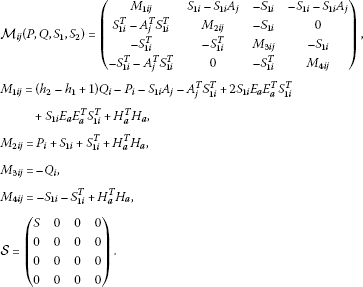The following theorem can be derived from Theorem 3.1.

Theorem 3.2 The nonlinear uncertain system (3.9) is robustly stabilizable by the delayed feedback control (3.10), where$\left(F\left(\xi \right)+\mathrm{\Delta }F\left(k\right)\right)={\left(B\left(\xi \right)+\mathrm{\Delta }B\left(k\right)\right)}^{T}{\left[\left(B\left(\xi \right)+\mathrm{\Delta }B\left(k\right)\right){\left(B\left(\xi \right)+\mathrm{\Delta }B\left(k\right)\right)}^{T}\right]}^{-1}$, if there exist symmetric matrices${P}_{i}>0$, ${Q}_{i}>0$, $i=1,2,\dots ,p$and constant matrices${S}_{1i}$, $i=1,2,\dots ,p$, $S\ge 0$satisfying the following LMIs:

1. (i)

${\mathcal{M}}_{ii}\left(P,Q,{S}_{1}\right)+\mathcal{S}<0$, $i=1,2,\dots ,p$.

2. (ii)

${\mathcal{M}}_{ij}\left(P,Q,{S}_{1}\right)+{\mathcal{M}}_{ji}\left(P,Q,{S}_{1}\right)-\frac{2}{p-1}\mathcal{S}<0$, $i=1,2,\dots ,p-1$; $j=i+1,\dots ,p$.

Proof Taking ${S}_{1i}={S}_{2i}$ and using the feedback control (3.10), the closed-loop nonlinear uncertain system becomes system (${\mathrm{\Sigma }}_{\xi }$), where $\left(D\left(\xi \right)+\mathrm{\Delta }D\left(k\right)\right)=\left(B\left(\xi \right)+\mathrm{\Delta }B\left(k\right)\right)\left(F\left(\xi \right)+\mathrm{\Delta }F\left(k\right)\right)=\left(B\left(\xi \right)+\mathrm{\Delta }B\left(k\right)\right){\left(B\left(\xi \right)+\mathrm{\Delta }B\left(k\right)\right)}^{T}{\left[\left(B\left(\xi \right)+\mathrm{\Delta }B\left(k\right)\right){\left(B\left(\xi \right)+\mathrm{\Delta }B\left(k\right)\right)}^{T}\right]}^{-1}=I$. Since ${S}_{1}\left(\xi \right)\left(D\left(\xi \right)+\mathrm{\Delta }D\left(k\right)\right)={S}_{1}\left(\xi \right)$, the robust stability condition of the closed-loop nonlinear uncertain system (3.11), by Theorem 3.1, is immediately derived. □

Remark 3.2 The stabilization conditions of Theorem 3.2 are more appropriate for practical systems since practically it is impossible to know exactly the delay but lower and upper bounds are always possible.

## 4 Numerical examples

To illustrate the effectiveness of the previous theoretical results, we consider the following numerical examples.

Example 4.1 (Robust stability)

Consider nonlinear uncertain system (${\mathrm{\Sigma }}_{\xi }$) for $p=2$, where the delay function $h\left(k\right)$ is given by

$h\left(k\right)=1+19{sin}^{2}\frac{k\pi }{2},\phantom{\rule{1em}{0ex}}k=0,1,2,\dots ,$

and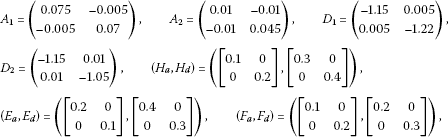with any time-varying delay function $h\left(k\right)$ with ${h}_{1}=1$, ${h}_{2}=20$. By using the LMI Toolbox in MATLAB, the LMIs (i) and (ii) of Theorem 3.1 are feasible with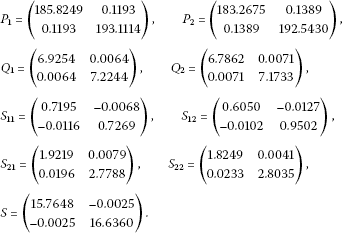Therefore, the nonlinear uncertain system is robustly stable.

Example 4.2 (Robust stabilization)

Consider nonlinear uncertain control system (3.9) for $p=2$, where the delay function $h\left(k\right)$ is given by

$h\left(k\right)=1+32{sin}^{2}\frac{k\pi }{2},\phantom{\rule{1em}{0ex}}k=0,1,2,\dots ,$

and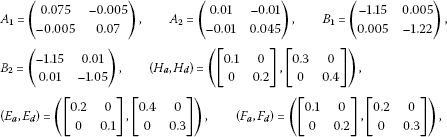with any time-varying delay function $h\left(k\right)$ with ${h}_{1}=1$, ${h}_{2}=33$, ${\xi }_{1}=0.5$, ${\xi }_{2}=0.5$. By using the LMI Toolbox in MATLAB, the LMIs (i) and (ii) of Theorem 3.2 are feasible withTherefore, the nonlinear uncertain system is robustly stabilizable with the feedback control

$\begin{array}{rcl}u\left(k\right)& =& {\left(B\left(\xi \right)+\mathrm{\Delta }B\left(k\right)\right)}^{T}{\left[\left(B\left(\xi \right)+\mathrm{\Delta }B\left(k\right)\right){\left(B\left(\xi \right)+\mathrm{\Delta }B\left(k\right)\right)}^{T}\right]}^{-1}x\left(k-h\left(k\right)\right)\\ =& {\left(0.5{B}_{1}+0.5{B}_{2}+\mathrm{\Delta }B\left(k\right)\right)}^{T}\left[\left(0.5{B}_{1}+0.5{B}_{2}\\ +\mathrm{\Delta }B\left(k\right)\right){\left(0.5{B}_{1}+0.5{B}_{2}+\mathrm{\Delta }B\left(k\right)\right)}^{T}{\right]}^{-1}x\left(k-h\left(k\right)\right)\\ =& \left(\begin{array}{cc}-0.8881& -0.0061\\ -0.0061& -0.9100\end{array}\right)x\left(k-h\left(k\right)\right).\end{array}$

Therefore, the feedback delayed controller is

$u\left(k\right)=\left[\begin{array}{c}-0.8881{x}_{1}\left(k-h\left(k\right)\right)-0.0061{x}_{2}\left(k-h\left(k\right)\right)\\ -0.0061{x}_{1}\left(k-h\left(k\right)\right)-0.9100{x}_{2}\left(k-h\left(k\right)\right)\end{array}\right].$

## 5 Conclusion

In this article, new delay-dependent robust stability conditions for nonlinear uncertain polytopic delay-difference equations with interval time-varying delays have been presented in terms of LMIs. An application in robust stabilization of nonlinear uncertain control discrete systems with time-delayed feedback controllers has been studied. Numerical examples have been given to demonstrate the effectiveness of the proposed conditions.

## References

1. Agarwal RP: Difference Equations and Inequalities. Dekker, New York; 2000.

2. Chen WH, Guan ZH, Lu X: Delay-dependent guaranteed cost control for uncertain discrete-time systems with delays. IEE Proc. Part D. Control Theory Appl. 2003, 150: 412–416. 10.1049/ip-cta:20030572

3. Elaydi S, Gyri I: Asymptotic theory for delay difference equations. J. Differ. Equ. Appl. 1995, 1: 99–116. 10.1080/10236199508808012

4. Kolmanovskii V, Myshkis A: Applied Theory of Functional Differential Equations. Springer, Berlin; 1992.

5. Mao WJ, Chu J: D -stability and D -stabilization of linear discrete-time delay systems with polytopic uncertainties. Automatica 2009, 45: 842–846. 10.1016/j.automatica.2008.11.003

6. Nam PT, Hien HM, Phat VN: Asymptotic stability of linear state-delayed neutral systems with polytope type uncertainties. Dyn. Syst. Appl. 2010, 19: 63–74.

7. Phat VN, Park JY: On the Gronwall’s inequality and stability of nonlinear discrete-time systems with multiple delays. Dyn. Syst. Appl. 2001, 1: 577–588.

8. Phat VN: Constrained Control Problems of Discrete Processes. World Scientific, Singapore; 1996.

9. Hsien TL, Lee CH: Exponential stability of discrete-time uncertain systems with time-varying delays. J. Franklin Inst. 1995, 322: 479–489.

10. Ji DH, Park JH, Yoo WJ, Won SC: Robust memory state feedback model predictive control for discrete-time uncertain state delayed systems. Syst. Control Lett. 2005, 54: 1195–1203. 10.1016/j.sysconle.2005.04.007

11. Phat VN, Bay NS: Stability analysis of nonlinear retarded difference equations in Banach spaces. Comput. Math. Appl. 2003, 45: 951–960. 10.1016/S0898-1221(03)00068-3

12. Boukas EK: State feedback stabilization of nonlinear discrete-time systems with time-varying delays. Nonlinear Anal. 2007, 66: 1341–1350. 10.1016/j.na.2006.01.020

13. Gao H, Chen T: New results on stability of discrete-time systems with time-varying delays. IEEE Trans. Autom. Control 2007, 52: 328–334.

14. Jiang X, Han QL, Yu X: Stability criteria for linear discrete-time systems with interval-like time-varying delays. Proceedings of the American Control Conference 2005, 2817–2822.

15. Phat VN, Nam PT: Exponential stability and stabilization of uncertain linear time-varying systems using parameter-dependent Lyapunov function. Int. J. Control 2007, 80: 1333–1341. 10.1080/00207170701338867

16. Phat VN, Ratchagit K: Stability and stabilization of switched linear discrete-time systems with interval time-varying delay. Nonlinear Anal. Hybrid Syst. 2011, 5: 605–612. 10.1016/j.nahs.2011.05.006

17. Ratchagit K: Asymptotic stability of nonlinear delay-difference system via matrix inequalities and application. Int. J. Comput. Methods 2009, 6: 389–397. 10.1142/S0219876209001899

18. Phat VN, Kongtham Y, Ratchagit K: LMI approach to exponential stability of linear systems with interval time-varying delays. Linear Algebra Appl. 2012, 436: 243–251. 10.1016/j.laa.2011.07.016

19. Ratchagit K, Phat VN: Stability criterion for discrete-time systems. J. Inequal. Appl. 2010., 2010:

20. Ratchagit K, Phat VN: Robust stability and stabilization of linear polytopic delay-difference equations with interval time-varying delays. Neural Parallel Sci. Comput. 2011, 19: 361–372.

21. Zhang B, Xu S, Zou Y: Improved stability criterion and its applications in delayed controller design for discrete-time systems. Automatica 2008, 44: 2963–2967. 10.1016/j.automatica.2008.04.017

22. Yu M, Wang L, Chu T: Robust stabilization of discrete-time systems with time-varying delays. Proceedings of the American Control Conference 2005, 3435–3440.

23. He Y, Wu M, She JH, Liu GP: Parameter-dependent Lyapunov functional for stability of time-delay systems with polytope-type uncertainties. IEEE Trans. Autom. Control 2004, 49: 828–832. 10.1109/TAC.2004.828317

24. Henrion D, Arzelier D, Peaucelle D, Sebek M: An LMI condition for robust stability of polynomial matrix polytopes. Automatica 2001, 37: 461–468. 10.1016/S0005-1098(00)00170-9

25. Coutinho DF, Fu M, Trofino A: Robust analysis and control for a class of uncertain nonlinear discrete-time systems. Syst. Control Lett. 2004, 53: 377–393. 10.1016/j.sysconle.2004.05.015

26. Kau SW, Liu Y, Hang L, Lee CH, Fang CH, Lee L: A new LMI condition for robust stability of discrete-time uncertain systems. Appl. Math. Comput. 2009, 215: 2035–2044. 10.1016/j.amc.2009.07.052

27. Kwon OM, Park JH: Exponential stability of uncertain dynamic systems including state delays. Appl. Math. Lett. 2006, 19: 901–907. 10.1016/j.aml.2005.10.017

## Acknowledgements

This work was supported by the Thai Research Fund Grant, the Higher Education Commission and Faculty of Science, Maejo University, Thailand. The authors thank anonymous reviewers for valuable comments and suggestions, which allowed us to improve the article.

## Author information

Authors

### Corresponding author

Correspondence to G Rajchakit.

### Competing interests

The authors declare that they have no competing interests.

### Authors’ contributions

The authors contributed equally and significantly in writing this article. The authors read and approved the final manuscript.

## Rights and permissions

Reprints and Permissions

Rajchakit, M., Rajchakit, G. LMI approach to robust stability and stabilization of nonlinear uncertain discrete-time systems with convex polytopic uncertainties. Adv Differ Equ 2012, 106 (2012). https://doi.org/10.1186/1687-1847-2012-106

• Accepted:

• Published:

• DOI: https://doi.org/10.1186/1687-1847-2012-106

### Keywords

• robust stability and stabilization
• nonlinear uncertain discrete-time systems
• convex polytopic uncertainties
• Lyapunov-Krasovskii functional
• linear matrix inequality